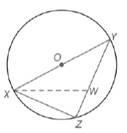Chapter 6.1, Problem 43EElementary Geometry For College St...

7th Edition
Alexander + 2 others
ISBN: 9781337614085

Solutions

Chapter
SectionElementary Geometry For College St...

7th Edition
Alexander + 2 others
ISBN: 9781337614085
Textbook Problem

In ⊙ O , O Y = 5 and X Z = 6. If X W ¯ ≅ W Y ¯ , find W Z .To determine

To calculate:

The WZ by using the provided figure.

Explanation

Given: In O, OY=5, and XZ=6 if XW¯WY¯

Pythagorean Theorem:

In a right triangle XYZ, (XY)2+(YZ)2=(XZ)2.

Calculation:

We need to find WZ.

Let WZ=y and x represent the measure of the congruent segments XW¯WY¯.

Since, OY=5 is a radius of circle O.

We know that, the diameter is double the radius of the circle.

Thus, XY=10.

Since, XYZ and XWZ are right triangles.

Thus, by using the Pythagorean theorem for XYZ triangle.

Still sussing out bartleby?

Check out a sample textbook solution.

See a sample solution

The Solution to Your Study Problems

Bartleby provides explanations to thousands of textbook problems written by our experts, many with advanced degrees!

Get Started

Solve the equations in Exercises 126. (x+1)2(x+2)3(x+1)3(x+2)2(x+2)6=0

Finite Mathematics and Applied Calculus (MindTap Course List)

Find limh01h22+h1+t3dt

Single Variable Calculus: Early Transcendentals, Volume I

What is the value of (X + 1) for the following scores: 0, 1, 4, 2? a. 8 b. 9 c. 11 d. 16

Essentials of Statistics for The Behavioral Sciences (MindTap Course List)